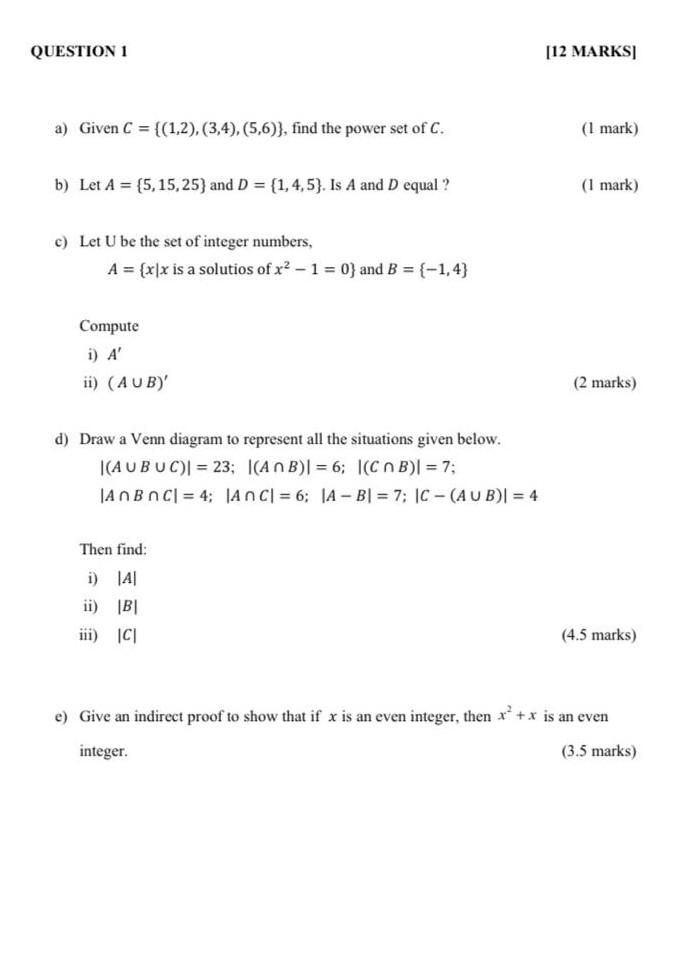### Create an Account

Already have account?

### Forgot Your Password ?

Home / Questions / QUESTION 1 [12 MARKS) a) Given C = {(1,2), (3,4), (5,6)), find the power set of C. (1 mark...

# QUESTION 1 [12 MARKS) a) Given C = {(1,2), (3,4), (5,6)), find the power set of C. (1 mark) b) Let A = {5,15,25) and D = (1,4,5). Is A and D equal ? (1 mark) c) Let U be the set of integer numbers, A

QUESTION 1 [12 MARKS) a) Given C = {(1,2), (3,4), (5,6)), find the power set of C. (1 mark) b) Let A = {5,15,25) and D = (1,4,5). Is A and D equal ? (1 mark) c) Let U be the set of integer numbers, A = {x|x is a solutios of x2 - 1 = 0} and B = {-1,4} Compute i) A' ii) (AUB)' (2 marks) d) Draw a Venn diagram to represent all the situations given below. |(AUB UC) = 23; (ANB) = 6; I(CAB) = 7; JAN BAC = 4; An C| = 6; A - Bl = 7; 1C - (AUB) = 4 Then find: i) A ii) IBI iii) C (4.5 marks) e) Give an indirect proof to show that if x is an even integer, then x + x is an even integer (3.5 marks)Apr 14 2021 View more View Less

#### Answer (Solved)Subscribe To Get Solution